# Always look on the light side of graphs

Consider a set of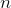distinct points in the plane, no three of them on the same line. Draw straight-line segments joining pairs of those points. This is called a geometric graph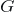and here we are going to focus on the segments of such a graph.

Every segment has two sides, defined by the line in which it is contained. Such a side is said to be a light side if it does not contain, totally, any other segment. Note that the sides are considered to be open, excluding the line.

Next, a segment is said to be a light segment if it has a light side. Finally, a geometric graph is a light graph if all of its segments are light segments.

In this figure, the red segment is light, because its upper side is light. Note that there is a segment sharing a point with the red one; since the sides exclude the line, that segment is not totally contained in the upper side. On the contrary, the blue segment is not light because both sides contain, totally, some segment and, therefore, the blue segment has no light side. Hence, the graph is not a light graph.

Now suppose that you are given a numberand you are asked to draw as many light segments as possible onpoints. How many light segments can you draw? In other words:

Which is the maximum possible number of segments in a light graph?

A bound is given by Ackerman et al. 1, who provide an easy-to-read proof showing that:

A light geometric graph onpoints has at most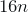segments.

The problem can be generalized, by allowing a certain number of segments to be contained in a light side. Hence, a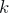-light side contains, totally, at mostsegments. Following the lines of the original problem, a segment is said to be a-light segment if it has a-light side. Finally, a geometric graph is a-light graph if all of its segments are-light segments.

Again, if you are given a numberand you want to draw as many-light segments as possible onpoints, how many can you draw? Equivalently:

Which is the maximum possible number of segments in a-light graph?

Ackerman et al. also show a bound for this generalized case, using the previous one (which, in fact, is that of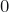-light graphs) together with a probabilistic argument. Their result says that:

A-light geometric graph onpoints has at most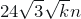segments.

Asymptotically this gives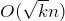segments, which is also shown to be the best answer possible. Considerpoints forming a regular polygon and connect with a segment those points whose cyclic distance is at most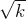. This gives a-light geometric graph with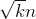edges.

The figure shows the case with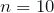and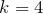, in which the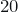edges are light.

It is interesting to know that the paper cited above extends a previous result by Ackerman and Pinchasi 2. There, they studied a stronger problem, considering general lines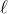instead of segments. A-light side of a line is defined in a similar manner and, thus,-light lines are those having a light side. A geometric graph is said to be a-near bipartite graph if every lineis a-light line with respect to the graph.

Since-light graphs are a particular case of-near bipartite graphs, in which only segments and not general linesare considered, the bound provided for-light graphs applies as well to-near bipartite graphs.

Before finishing, let us mention another concept related to that of light segments. Two segments are said to be avoiding segments if no line containing one of them intersects the other. Equivalently, two segments are avoiding if they are opposite edges of a convex quadrilateral.

The figure shows a pair of avoiding segments (left) and a pair of segments which are not avoiding (right). For a connection between this notion and the previous one, observe that a light segment has a side not containing segments and, hence, it can only avoid those segments totally contained in the heavy side (or should we call it the dark side?)

The notion of avoiding segments was introduced by Kupitz 3, who conjectured that:

Any geometric graph onpoints which does not contain a pair of avoiding segments has at most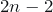edges.

The conjecture was proved to be true some years later by Valtr 4. The techniques used there can be applied, as well, to deduce an upper bound for the maximum number of segments in a light geometric graph. Nevertheless, the constant obtained in that way is different than the one showed by Ackerman et al.

Finally, let me finish by encouraging you to explore this notions by making your own drawings. For instance, draw some set ofpoints and try to draw on it as many light segments as possible. And, remember, always look on the light side!

## References

1. Eyal Ackerman, Jacob Fox, Rom Pinchasi. A note on light geometric graphs. Discrete Mathematics 313 (2013) 1281-1283. DOI: http://dx.doi.org/10.1016/j.disc.2013.03.001
2. Eyal Ackerman, Rom Pinchasi. On the light side of geometric graphs. Discrete Mathematics 312 (2012) 1213-1217. DOI: http://dx.doi.org/10.1016/j.disc.2011.12.009
3. Yakov Shimeon Kupitz. On pairs of disjoint segments in convex position in the plane. Annals of Discrete Mathematics 20 (1984) 203-208. DOI: http://dx.doi.org/10.1016/S0304-0208(08)72827-5
4. Pavel Valtr. On geometric graphs with nopairwise parallel edges. Discrete and Computational Geometry 19 (3) (1998) 461-469. DOI: http://dx.doi.org/10.1007/PL00009364